# HOMER Pro 3.10Type: Input Variable Units: m Symbol: z

The altitude is the elevation above mean sea level. Altitude affects air density, which in turn affects wind turbine output. Therefore, HOMER considers the altitude when calculating the output of the wind turbine.

According to the ideal gas law, air density is determined by the following equation: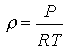where: ρ = air density [kg/m3] P = pressure [Pa] R = gas constant [287 J/kgK] T = temperature [K]

The quantity that HOMER uses is the air density ratio, which is the actual air density divided by the air density under standard conditions (sea level, 15 degrees Celsius). When calculating the output of the wind turbine at the specified altitude, HOMER multiplies the power output obtained from the wind turbine power curve by the air density ratio. Using the ideal gas law, the air density ratio can be expressed as follows: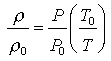where: P0 = standard pressure [101,325 Pa] T0 = standard temperature [288.16 K]

Altitude affects both pressure and temperature. The US Standard Atmosphere uses the simplifying assumption that, up to an altitude of 11,000m, temperature decreases linearly with altitude according to the following equation: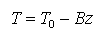where: B = lapse rate [0.00650 K/m] z = altitude [m]

Using the assumption that temperature decreases linearly with altitude, the air pressure can be shown to depend on the altitude according to the following equation: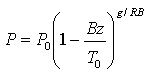where: g = gravitational acceleration [9.81 m/s2]

By substituting these equations for P and T into the equation defining the air density ratio, we get the following equation for the air density ratio:On the right side of the equation above, only z, the altitude, is not constant. So, with the assumptions we have used, the air density ratio is a function of altitude alone. We can use this equation to produce a graph of air density ratio versus altitude, as shown below: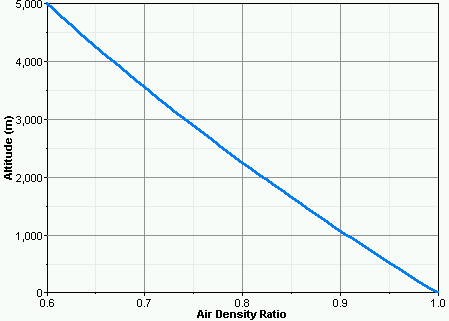The graph shows that at an altitude of 2000m, the air density ratio is about 0.82, meaning that air at that altitude is 82% as dense as air at standard temperature and pressure.